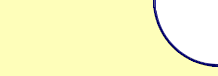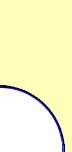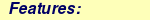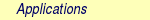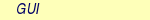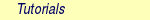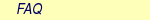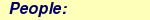MOLCAS manual:Next: 6.8 CASPT2 Up: 6. Program Based Tutorials Previous: 6.6 MBPT2

Subsections

# 6.7 RASSCF -- A Multi Configurational Self-Consistent Field Program

One of the central codes in MOLCAS is the RASSCF program, which performs multiconfigurational SCF calculations. Both Complete Active Space (CASSCF) and Restricted Active Space (RASSCF) SCF calculations can be performed with the RASSCF program module . An open shell Hartree-Fock calculation is not possible with the SCF but it can be performed using the RASSCF module. An input listing for a CASSCF calculation of water appears in Figure 6.7. RASSCF requires orbital information of the system which can be obtained in two ways. The LUMOrb indicates that the orbitals should be taken from a user defined orbital file, which is copied to the internal file INPORB. If this keyword is not given, the program will look for orbitals on the runfile in the preference order: RASORB, SCFORB and GUESSORB&RASSCF
Title=  The  CASSCF  energy  of  water  is  calculated  using  C2v  symmetry.  2  3B2  state.
nActEl=  8  0  0
Inactive=  1  0  0  0;  Ras2=  3  2  0  1
Symmetry=  2;  Spin=  3
CIRoot=  1  2;  2
LumOrb

The TITLe performs the same function as in the previous MOLCAS modules. The keyword INACtive specifies the number of doubly occupied orbitals in each symmetry that will not be included in the electron excitations and thus remain doubly occupied throughout the calculation. A diagram of the complete orbital space available in the RASSCF module is given in Figure 6.8.

In our calculation, we have placed the oxygen 1s orbital in the inactive space using the INACtive keyword. The keyword FROZen can be used, for example, on heavy atoms to reduce the Basis Set Superposition Error (BSSE). The corresponding orbitals will then not be optimized. The RAS2 keyword specifies the number of orbitals in each symmetry to be included in the electron excitations with all possible occupations allowable. Because the RAS1 and RAS3 spaces are zero (not specified in the input in Figure 6.7) the RASSCF calculation will produce a CASSCF wave function. The RAS2 space is chosen to use all the orbitals available in each symmetry (except the oxygen 1s orbital). The keyword NACTel specifies the number of active electrons (8), maximum number of holes in the Ras1 space (0) and the maximum number of electrons in the Ras3 space (0). Using the keywords RAS1 and/or RAS3 to specify orbitals and specifying none zero numbers of holes/electrons will produce a RASSCF wave function.We are, therefore, performing an 8in6 CASSCF calculation of water.

 Number of holes Number of electrons Description in RAS1 orbitals RAS2 orbitals in RAS3 orbitals SD-CI 2 0 2 SDT-CI 3 0 3 SDTQ-CI 4 0 4 Multi Reference SD-CI 2 n 2 Multi Reference SD(T)-CI 3 n 2

There are a number of wave function types that can be performed by manipulating the RAS1 and RAS3 spaces. Table 6.2 lists a number of types obtainable. The first three are Configuration Interaction (CI) wave functions of increasing magnitude culminating with a Single, Double, Triples and Quadruples (SDTQ) CI. These can become multi reference if the number of RAS2 orbitals is non-zero. The last type provides some inclusion of the triples excitation by allowing three holes in the RAS1 orbitals but save computation cost by only allowing double excitations in the RAS3 orbitals.The symmetry of the wave function is specified using the SYMMetry keyword. It specifies the number of the symmetry subgroup in the calculation. We have chosen the second symmetry species, b2, for this calculation. We have also chosen the triplet state using the keyword SPIN. The keyword CIROot has been used to instruct RASSCF to find the second excited state in the given symmetry and spin. This is achieved by specifying the number of roots, 1, the dimension of the small CI matrix which must be as large as the highest required root and the number of the required second root. Only for averaged calculations CIROot needs an additional line containing the weight of the selected roots (unless equal weights are used for all states).

As an alternative to giving inactive and active orbital input we can use the type index input on the INPORB and indicate there which type the different orbitals should belong to: frozen (f), inactive (i), RAS1 (1), RAS2 (2), RAS3 (3), secondary (s), or deleted (d). This approach is very useful when the input orbitals have been run through LUSCUS, which is used to select the different subspaces. LUSCUS will relabel to orbitals according to the users instructions and the corresponding orbital file ,GvOrb can be linked as the INPORB in the RASSCF program without any further input.

A level shift was included using the LEVShift keyword to improve convergence of the calculation. In this case, the calculation does not converge without the use of the level shift. It is advisable to perform new calculations with a non-zero LEVShift value (the default value is 0.5). Another possibility is to increase the maximum number of iterations for the macro and the super-CI Davidson procedures from the default values (200,100) using the keyword ITERations.

Sometimes convergence problems might appear when the wave function is close to fulfill all the convergence criteria. An infrequent but possible divergence might appear in a calculation starting from orbitals of an already converged wave function, or in cases where the convergence thresholds have been decreased below the default values. Option TIGHt may be useful in those cases. It contains the thresholds criteria for the Davidson diagonalization procedure. In situations such as those described above it is recommended to decrease the first parameter of TIGHt to a value lower than the default, for instance 1.0d-06.

## 6.7.1 RASSCF Output

The RASSCF section of the MOLCAS output contains similar information to the SCF output. Naturally, the fact that we have requested an excited state is indicated in the output. In fact, both the lowest triplet state and the first excited state or second root are documented including energies. For both of these states the CI configurations with a coefficient greater than 0.05 are printed along with the partial electron distribution in the active space. Figure 6.9 shows the relevant output for the second root calculated. There are three configurations with a CI-coefficient larger than 0.05 and two with very much larger values. The number of the configuration is given in the first column and the CI-coefficient and weight are given in the last two columns. The electron occupation of the orbitals of the first symmetry for each configuration is given under the 111' using 2' for a fully occupied orbital and u' for a singly occupied orbital containing an electron with an up spin. The down spin electrons are represented with a d'. The occupation numbers of the active space for each symmetry is given below the contributing configurations. It is important to remember that the active orbitals are not ordered by any type of criterion within the active space.printout of CI-coefficients larger than   .05 for root   2
energy=    -75.443990
conf/sym  111 22 4     Coeff  Weight
3  22u u0 2    .64031  .40999
4  22u 0u 2    .07674  .00589
13  2u0 2u 2   -.75133  .56450
14  2u0 u2 2    .06193  .00384
19  udu 2u 2    .06489  .00421

Natural orbitals and occupation numbers for root  2
sym 1:   1.986957   1.416217    .437262
sym 2:   1.567238    .594658
sym 4:   1.997668


The molecular orbitals are displayed in a similar fashion to the SCF section of the output except that the energies of the active orbitals are not defined and therefore are displayed as zero and the electron occupancies are those calculated by the RASSCF module. In a state average calculation (more than one root calculated), the MOs will be the natural orbitals corresponding to the state averaged density matrix (called pseudo-natural orbitals) and the occupation numbers will be the corresponding eigenvalues. Natural orbital occupation numbers for each state are printed as shown in Figure 6.9, but the MOs specific to a given state are not shown in the output. They are, however, available in the JOBIPH file. A number of molecular properties are also computed for the requested electronic state in a similar fashion to the SCF module.

## 6.7.2 Storing and Reading RASSCF Orbitals and Wave Functions

Part of the information stored in the RASSCF output file, JOBIPH, for instance the molecular orbitals and occupation numbers can be also found in an editable file named RASORB, which is automatically generated by RASSCF. In case more than one root is used the natural orbitals are also stored in files RASORB.1, RASORB.2, etc, up to ten. In such cases the file RASORB contains the averaged orbitals. If more roots are used the files can be generated using the OUTOrbitals keyword. The type of orbital produced can be either AVERaged, NATUral, CANOnical or SPIN (keywords) orbitals. The OUTOrbitals keyword, combined with the ORBOnly keyword, can be used to read the JOBIPH file and produce an orbital file, RASORB, which can be read by a subsequent RASSCF calculation using the same input section. The formatted RASORB file is useful to operate on the orbitals in order to obtain appropriate trial orbitals for a subsequent RASSCF calculation. In particular the type index can be changed directly in the file if the RASSCF program has converged to a solution with wrong orbitals in the active space. The RASSCF program will, however, automatically place the orbital files from the calculation in the user's home directory under the name $Project.RasOrb, etc. In calculations with spin different from zero the program will also produce the spin orbital files$Project.SpdOrb1, etc for each state. These orbitals can be used by the program LUSCUS to produce spin densities.

## 6.7.3 RASSCF - Basic and Most Common Keywords

 Keyword Meaning SYMMetry Symmetry of the wave function (according to GATEWAY) (1 to 8) SPIN Spin multiplicity CHARGE Molecular charge NACTel Three numbers: Total number of active electrons, holes in Ras1, particles in Ras3 INACtive By symmetry: doubly occupied orbitals RAS1 By symmetry: Orbitals in space Ras1 (RASSCF) RAS2 By symmetry: Orbitals in space Ras1 (CASSCF and RASSCF) RAS3 By symmetry: Orbitals in space Ras1 (RASSCF) CIROot Three numbers: number of CI roots, dimension of the CI matrix, relative weights (typically 1) LUMORB/FILEORB use definition of active space from Orbital file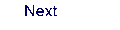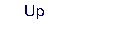Next: 6.8 CASPT2 Up: 6. Program Based Tutorials Previous: 6.6 MBPT2